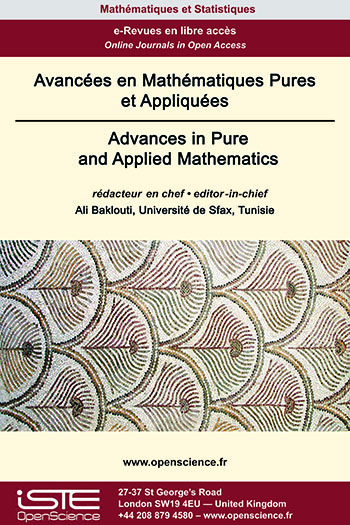# Vol 13 - Issue 3 (June 2022)

## List of Articles

Limit sets and global dynamic for 2-D divergence-free vector fields

The global structure of divergence-free vector fields on closed surfaces is investigated. We prove that if M is a closed surface and ${V}$ is a divergence-free $C^{1}$-vector field with finitely many singularities on M then every orbit L of $\mathcal{V}$ is one of the following types: (i) a singular point, (ii) a periodic orbit, (iii) a closed (non periodic) orbit in M* = M - Sing($\mathcal{V}$), (iv) a locally dense orbit, where Sing($\mathcal{V}$) denotes the set of singular points of $\mathcal{V}$. On the other hand, we show that the complementary in M of periodic components and minimal components is a compact invariant subset consisting of singularities and closed (non compact) orbits in M*. These results extend those of T. Ma and S. Wang in [Discrete Contin. Dynam. Systems, 7 (2001), 431-445] established when the divergence-free vector field $\mathcal{V}$ is regular that is all its singular points are non-degenerate.

Weighted estimates for operators associated to the Bergman-Besov kernels

We characterize the weights for which we have the boundedness of standard weighted integral operators induced by the Bergman-Besov kernels acting between two weighted Lebesgue classes on the unit ball of ℂN in terms of Békollè - Bonami type condition on the weights. To accomplish this we employ the proof strategy originated by Békollè.

A result on Bruck Conjecture related to Shift Polynomials

This paper mainly concerns about establishing the Bruck conjecture for differential-difference polynomial generated by an entire function. The polynomial considered is of finite order and involves the entire function $f(z)$ and its shift $f(z + c)$ where $c \in ℂ$. Suitable examples are given to prove the sharpness of sharing exceptional values of Borel and Nevanlinna.

Existence Results for Singular p(x)-Laplacian Equation

This paper is concerned with the existence of solutions for the following class of singular fourth order elliptic equations
$\left\{ \begin{array}{ll} \Delta\Big(｜x｜^{p(x)}｜\Delta u｜^{p(x)-2}\Delta u\Big)=a(x)u^{-\gamma (x)}+\lambda f(x,u),\quad \mbox{in }\Omega, \\ u=\Delta u=0, \quad \mbox{on }\partial\Omega. \end{array} \right.$
where $\Omega$ is a smooth bounded domain in $\mathbb{R}^N, \gamma :\overline{\Omega}\rightarrow (0,1)$ be a continuous function, $f\in C^{1}( \overline{\Omega}\times \mathbb{R}), p:\; \overline{\Omega}\longrightarrow \;(1,\infty)$ and $a$ is a function that is almost everywhere positive in $\Omega$. Using variational techniques combined with the theory of the generalized Lebesgue-Sobolev spaces, we prove the existence at least one nontrivial weak solution.### Other issues :

2020

Volume 20- 11

Issue 1 (May 2020)
Issue 2 (September 2020)

2021

Volume 21- 12

Issue 1 (January 2021)
Issue 2 (May 2021)
Issue 3 (Special AUS-ICMS 2020)
Issue 4 (September 2021)

2022

Volume 22- 13

Forthcoming papers

Issue 1 (January 2022)
Issue 2 (March 2022)
Issue 3 (June 2022)